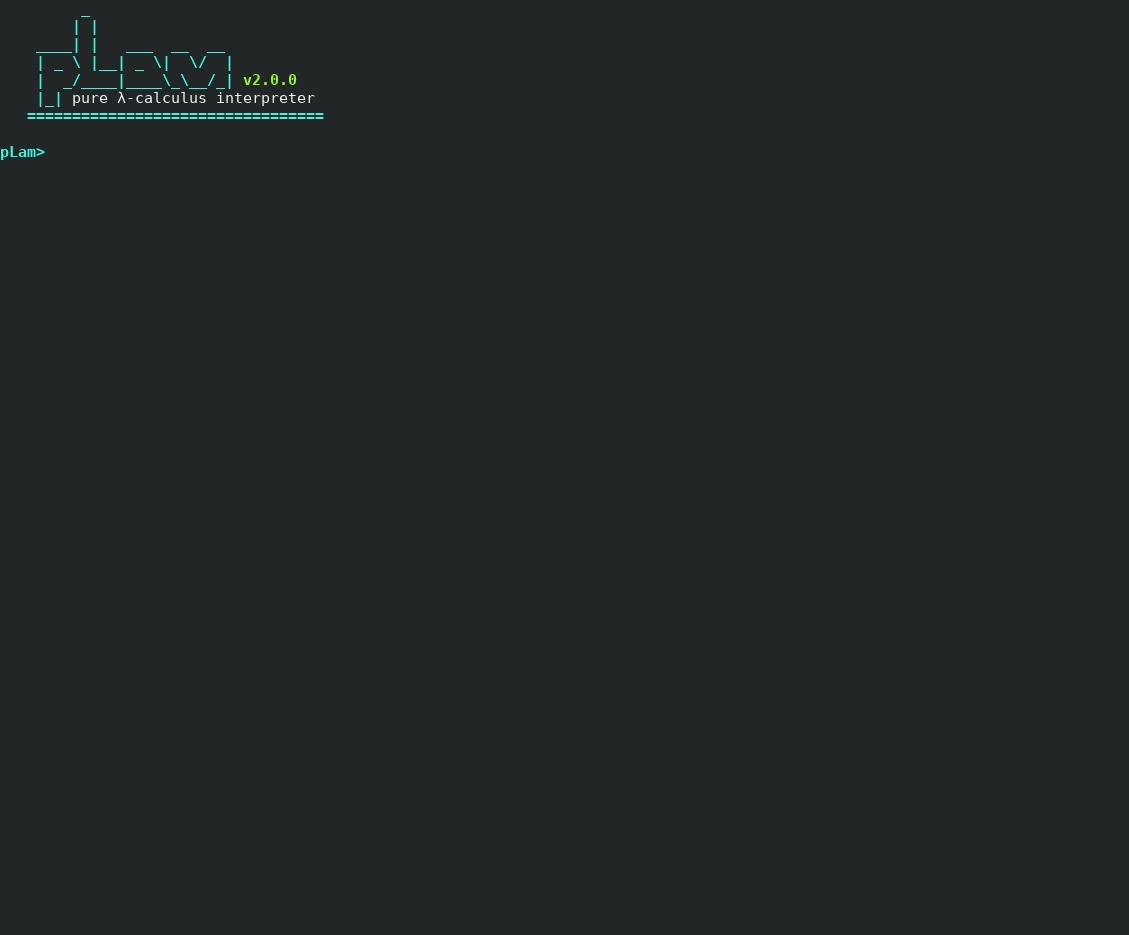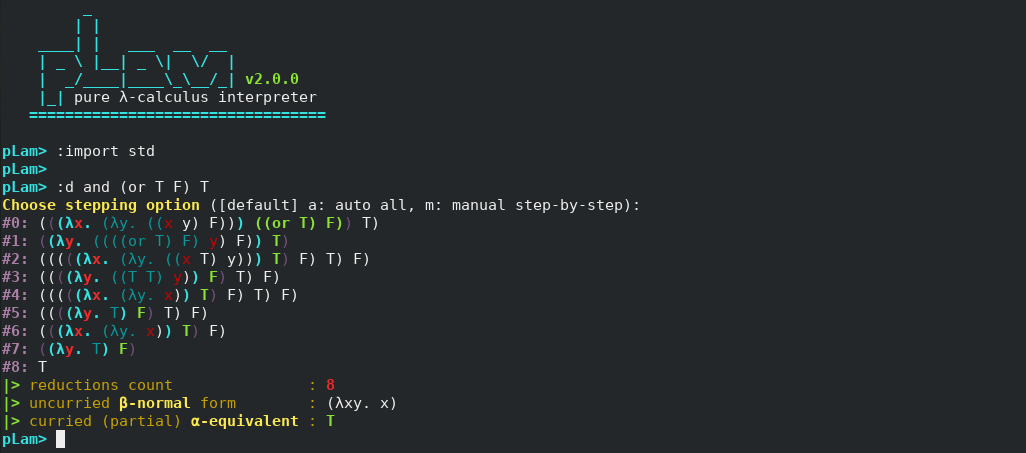Need help with pLam?
Click the “chat” button below for chat support from the developer who created it, or find similar developers for support.#### About the developerslovnicki386 Stars17 ForksMIT License268 Commits4 Opened issues#### Description

An interpreter for learning and exploring pure λ-calculus!
?

#### Need anything else?#### Contributors list# 197,392
Dart
Haskell
Shell
lambda
244 commits# 551,262
Haskell
Shell
lambda
lambda-...
6 commits# 151,212
Haskell
Shell
jvm-lan...
compile...
4 commits# 644,333
Haskell
Shell
lambda
lambda-...
1 commitpLam (pure Lambda calculus) is a tool to explore, define and evaluate various λ-expressions. Code written in pLam can be executed interactively within pLam's shell or stored in a file with

`.plam`
extension and run anytime.

Inside

`import/`
directory, many useful λ-expressions are already implemented to be used as libraries.

# Install a Package

### Arch Linux

pLam's AUR package is at https://aur.archlinux.org/packages/plam thanks to @Xmgplays.

Git Clone URL: https://aur.archlinux.org/plam.git

(coming soon...)

# Build from source

## Prerequisites

This project builds using Haskell tool stack documented at https://docs.haskellstack.org/en/stable/README/.

On most Unix systems, you can get stack by typing:

```curl -sSL https://get.haskellstack.org/ | sh
```
or:
```wget -qO- https://get.haskellstack.org/ | sh
```
On Windows, you can download 64-bit installer given at https://docs.haskellstack.org/en/stable/README/.

## First time setup

1. clone project repository
```git clone https://github.com/sandrolovnicki/pLam.git
```
2. go to project directory
```cd pLam
```
3. setup stack on isolated location
```stack setup
```

## Building

1. use stack to build project
```stack build
```
note: if build was not successful, it may be due to:
• curses library
• the solution is to install it (on Ubuntu:
`sudo apt-get install libncurses5-dev libncursesw5-dev`
)

## Running (locally)

5.a) use stack to run project executable from project's directory

```stack exec plam
```

## Running (globally (Unix systems))

5.b) use

`make_global.sh`
script to create a global command 'plam' that can be used to start pLam from anywhere in your system. The script will also change your import path in src/Config.hs so you need to build the project again.
```sudo ./make_global.sh
stack build
```
Now, (and anytime in the future!), you can start pLam from anywhere in your system by just typing ``` plam

# Syntax and semantics

## λ-expressions

### Variable

λ-variable is required to be lowercase and a single letter. For example,

`x`
is a good λ-variable for pLam and
`X`
,
`var`
,... are not. There are also environment variables (names for defined λ-expressions) which are every string that is not parsed as λ-variable, λ-abstraction or λ-application.

### Abstraction

λ-abstraction is written the same as in the language of pure (untyped) λ-calculus, except that pLam treats a symbol

`\`
as
`λ`
and it is required to write a space after
`.`
. For example,
`λx.λy.x`
would be written
`\x. \y. x`
in pLam. One can also write λ-abstraction in the "uncurried" form:
`\xy. x`
or
`\x y. x`
.

### Application

λ-application is written like 2 λ-expressions separated by a space, for example

`(\x. x) (\xy.x)`
or
`(\x. x) MyExpression`
or
`myexp1 myexp2`
. Brackets
`(`
and
`)`
are used as usual and are not required to be written for application association; the default association is to the left, so
`M N P`
is parsed as
`(M N) P`
and one only needs to specify with brackets if the intended expression should be
`M (N P)`
.

## Commands

A block of code in pLam is a line, and possible lines (commands) are the following:

### Define

• syntax:
` = `
• semantics: let the
be a name for
.
• examples:
`T = \x y. x`
,
`myexpression = T (T (\x. x) T) T`
• restriction:
needs to be of length>1 or starting with uppercase letter

### Evaluate

• syntax:
`? ? `
where
s are evaluation options;
`:d`
and/or
`:cbv`
• semantics: reduce the
to β-normal form. If
`:d`
is chosen as option, all the reduction steps will be shown. If
`:cbv`
is chosen as option, reductions will be performed in a call-by-value manner, first reducing the argument before substituting it for bound variable. That is, call-by-name is the default reduction option if
`:cbv`
is not chosen.
• example:
`\x y. x`
,
`:d T (T (\x. x) T) T`
,
`:d :cbv T (T (\x. x) T) T`
,
`:cbv and (or T F) T`
• example 2:
`F omega T`
will reduce to
`T`
, but
`:cbv F omega T`
will run forever
• restriction: none

### Import

• syntax:
`:import `
• semantics: put all the expressions defined in the file
`import/.plam`
into the list of environment variables.
• example:
`:import std`
• restriction:
`.plam`
has to be inside
`import/`
directory within the pLam project directory

### Export

• syntax
`:export `
• semantics: put all the expressions in the list of environment variables into the file
`import/.plam`
• example:
`:export test`
• restriction:
`.plam`
cannot already exist

### Comment

• syntax:
`--`
• semantics: a comment line
• example:
`-- this is a comment`
• restriction: none

### Run

• syntax:
`:run `
• semantics: runs a
`.plam`
file with relative path
`.plam`
• example:
`:run /examples/2.5.2`
• restrictions:
`~`
for home does not work

### Print

• syntax:
`:print `
• semantics: prints
to a new line. It mostly makes sense to use it in .plam programs to be executed, not in interactive mode where a comment should do the job better.
• example:
`:print this is a message`
• restrictions: none

## Syntactic Sugars

pLam is equipped with some (optional) shortcuts to work with often used expressions.

### Church numerals

Church numerals can be typed as

`0`
,
`1`
,
`2`
,... and pLam parses those integers as
`λfx. x`
,
`λfx. f x`
,
`λfx. f (f x)`
, ...

### Binary numerals

Similar to handling Church numerals, pLam also handles binary numerals from

`binary.plam`
library. You can type them as
`0b`
,
`1b`
,
`2b`
, ... which is them parsed as
`λp. p (λxy. y) (λexy.x)`
,
`λp. p (λxy. x) (λexy.x)`
,
`λp. p (λxy. y) (λp. p (λxy. x) (λexy.x))`
, ...
Note that binary numerals are nothing standard, but something I implemented, so I suppose the only documentation for them is here.

### Lists

List encoding is pretty standard;

`empty = T`
,
`append = λhtfl. l h t`
, and you can use syntact sugar which parses
`[1,2]`
into
`λfl. l 1 (λfl. l 2 empty)`
,
`[T,\x.x]`
into
`λfl. l T (λfl. l (λx.x) empty)`
and so on...

# Examples

NOTE: Output might be slightly different due to constant fixes and changes. Fully updated examples will be put each time they diverge too far from current.
All the examples can be found in

`examples/`
directory.

### Fun with booleans

```pLam> :import booleans
pLam>
pLam> and (or F (not F)) (xor T F)
|> reductions count               : 18
|> uncurried β-normal form        : (λxy. x)
|> curried (partial) α-equivalent : T
```

### Fun with arithmetic

```pLam> :import std
pLam>
pLam> mul (add 2 (S 2)) (sub (exp 2 3) (P 8))
|> reductions count               : 762
|> uncurried β-normal form        : (λfx. f (f (f (f (f x)))))
|> curried (partial) α-equivalent : 5
```

### Factorial

#### "standard" way

```pLam> :import std
pLam>
pLam> fFact = \f. \x. (isZ x) 1 (mul x (f (P x)))
pLam> Fact = Y fFact
pLam>
pLam> Fact 3
|> reductions count               : 646
|> uncurried β-normal form        : (λfx. f (f (f (f (f (f x))))))
|> curried (partial) α-equivalent : 6
```

#### primitive recursive way

```pLam> :import std
pLam> :import comp
pLam>
pLam> fact = PR0 1 (C22 mul (C2 S I12) I22)
pLam> fact 3
|> reductions count               : 898
|> uncurried β-normal form        : (λfx. f (f (f (f (f (f x))))))
|> curried (partial) α-equivalent : 6
```

### Binary numerals

```pLam> :import binary
pLam>
pLam> 0b
|> reductions count               : 2
|> uncurried β-normal form        : (λp.((p (λxy. y)) (λexy.x)))
|> curried (partial) α-equivalent : 0b
pLam>
pLam> 2048b
|> reductions count               : 24
|> uncurried β-normal form        : (λp.((p (λxy. y)) (λp.((p (λxy. y)) (λp.((p (λxy. y)) (λp.((p (λxy. y)) (λp.((p (λxy. y)) (λp.((p (λxy. y)) (λp.((p (λxy. y)) (λp.((p (λxy. y)) (λp.((p (λxy. y)) (λp.((p (λxy. y)) (λp.((p (λxy. y)) (λp.((p (λxy. x)) (λexy.x)))))))))))))))))))))))))
|> curried (partial) α-equivalent : (λp. ((p F) 1024b))
pLam>
pLam> addB 7b (subBs 2b 3b)
|> reductions count               : 9458
|> uncurried β-normal form        : (λp.((p (λxy. x)) (λp.((p (λxy. x)) (λp.((p (λxy. x)) (λexy.x)))))))
|> curried (partial) α-equivalent : 7b
```

### Lists

```pLam> :import list
pLam>
pLam> list = Merge [3,1] 
pLam> rlist = Reverse list
pLam>
pLam> Get 0 rlist
|> reductions count               : 243
|> uncurried β-normal form        : (λfx. f (f x))
|> curried (partial) α-equivalent : 2
pLam> Get 0 list
|> reductions count               : 50
|> uncurried β-normal form        : (λfx. f (f (f x)))
|> curried (partial) α-equivalent : 3
pLam>
pLam> QSort list
|> reductions count               : 459
|> uncurried β-normal form        : (λfl. (l (λfx. f x)) (λfl. (l (λfx. f (f x))) (λfl. (l (λfx. f (f (f x)))) (λfl. f))))
|> curried (partial) α-equivalent : (λf. (λl. ((l 1) (λf. (λl. ((l 2) (λf. (λl. ((l 3) empty)))))))))

```

### Redex coloring### Running the existing program:

```pLam> :run examples/2.5.2
=================================
< zero
=================================
|> reductions count               : 114
|> uncurried β-normal form        : (λfx. f (f x))
|> curried (partial) α-equivalent : 2
```

#### Without entering pLam's shell:

```plam ~/Projects/pLam/examples/2.5.2.plam
=================================
< zero
=================================
|> reductions count               : 114
|> uncurried β-normal form        : (λfx. f (f x))
|> curried (partial) α-equivalent : 2
Done.
```

#### Disclaimer for Haskell experts

I am not a Haskell expert. In fact, this is my first and only Haskell project. It is inevitable that existing code could be written better and I wish to do it in the upcoming future.
The goal of the project was to create an environment for easy building of new expressions from previously defined ones, so that one could explore λ-calculus. It was a helper tool so I could define and test a new numeral system in λ-calculus, for my master thesis. Now, when this all went well, the time is coming for me to get back to Haskell code.

### Contributing

If you would like to see some improvements or new features, you can open an issue. I will sort issues into milestones and you will know pretty quickly when to expect it to be done. If you can implement your ideas yourself, great! Pull requests are welcome.

We use cookies. If you continue to browse the site, you agree to the use of cookies. For more information on our use of cookies please see our Privacy Policy.